# Canny检测算法与实现

2019/04/10 10:10

## 1、原理

Canny 边缘检测算法是John F. Canny 于1986年开发出来的一个多级边缘检测算法，也被很多人认为是边缘检测的 最优算法, 最优边缘检测的三个主要评价标准是:

Canny算子求边缘点具体算法步骤如下：

1. 用高斯滤波器平滑图像．

2. 用一阶偏导有限差分计算梯度幅值和方向.

3. 对梯度幅值进行非极大值抑制.

4. 用双阈值算法检测和连接边缘．

## 2、实现步骤

2.1、消除噪声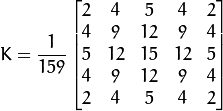2.2、计算梯度幅值和方向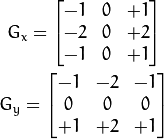在vs中可以看到sobel像素值和形状：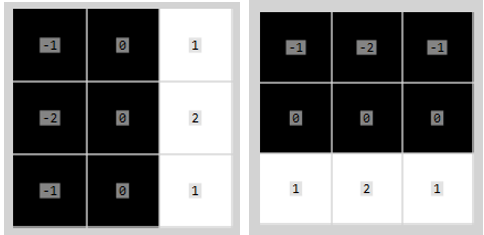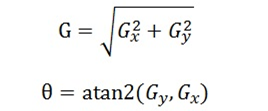2.3、非极大值抑制

1）  0：左边 和 右边

2） 45：右上 和 左下

3） 90：上边 和 下边

4）135：左上 和 右下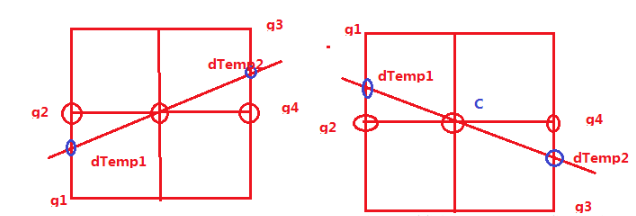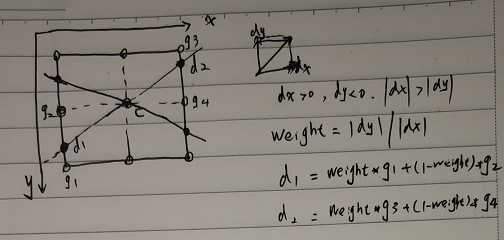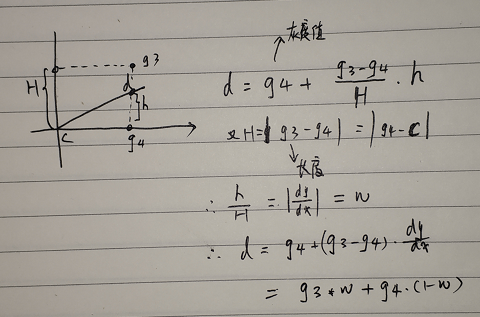Matlab使用非常有技巧的方式来计算方向，如下不仅做了dx、dy的大小判断还做了方向的判定。

witch direction
case 1
idx = find((iy<=0 & ix>-iy)  | (iy>=0 & ix<-iy));
case 2
idx = find((ix>0 & -iy>=ix)  | (ix<0 & -iy<=ix));
case 3
idx = find((ix<=0 & ix>iy) | (ix>=0 & ix<iy));
case 4
idx = find((iy<0 & ix<=iy) | (iy>0 & ix>=iy));
end

2.4、双阈值检测和区域连通

## 3、代码实现

3.1 计算梯度

/*
* Sobel 梯度计算
*/
{
int W = img.cols;
int H = img.rows;

Mat dx = Mat_<int>(img.size());
int border = (int)sobel.rows / 2;

for (int r = border; r < H - border; r++)
{
for (int c = border; c < W - border; c++)
{
float tmp = 0;
for (int i = -border; i <= border; i++) {
for (int j = -border; j <= border; j++) {
tmp += (int)img.data[(r + i)*W + c + j] * sobel.at<int>(i + border, j + border);
}
}

dx.at<int>(r, c) = tmp;
}
}
return dx;
}

3.2计算非极大值抑制（详细推导过程见参考文献文章）

/*
fucntion: non-maximum suppression
input:
pMag:   pointer to Magnitude,
sz: size of pMag (width = size.cx, height = size.cy)
limit: limitation
output:
pNSRst: result of non-maximum suppression
*/
void NonMaxSuppress(int *pMag, int * pGradX, int *pGradY, Size sz, int *pNSRst)
{
long x, y;
int nPos;
// the component of the gradient
int gx, gy;
// the temp varialbe
int g1, g2, g3, g4;
double weight;
double dTemp, dTemp1, dTemp2;
//设置图像边缘为不可能的分界点
for (x = 0; x < sz.width; x++)
{
pNSRst[x] = 0;
pNSRst[(sz.height - 1)*sz.width + x] = 0;
}
for (y = 0; y < sz.height; y++)
{
pNSRst[y*sz.width] = 0;
pNSRst[y*sz.width + sz.width - 1] = 0;
}

for (y = 1; y < sz.height - 1; y++)
{
for (x = 1; x < sz.width - 1; x++)
{
nPos = y * sz.width + x;
// if pMag[nPos]==0, then nPos is not the edge point
if (pMag[nPos] == 0)
{
pNSRst[nPos] = 0;
}
else
{
// the gradient of current point
dTemp = pMag[nPos];
// x,y 方向导数
//如果方向导数y分量比x分量大，说明导数方向趋向于y分量
if (abs(gy) > abs(gx))
{
// calculate the factor of interplation
weight = fabs(gx) / fabs(gy);
g2 = pMag[nPos - sz.width];  // 上一行
g4 = pMag[nPos + sz.width];  // 下一行
//如果x,y两个方向导数的符号相同
//C 为当前像素，与g1-g4 的位置关系为：
//g1 g2
//   C
//   g4 g3
if (gx*gy > 0)
{
g1 = pMag[nPos - sz.width - 1];
g3 = pMag[nPos + sz.width + 1];
}
//如果x,y两个方向的方向导数方向相反
//C是当前像素，与g1-g4的关系为：
//    g2 g1
//    C
// g3 g4
else
{
g1 = pMag[nPos - sz.width + 1];
g3 = pMag[nPos + sz.width - 1];
}
}
else
{
//插值比例
weight = fabs(gy) / fabs(gx);
g2 = pMag[nPos + 1]; //后一列
g4 = pMag[nPos - 1];    // 前一列
//如果x,y两个方向的方向导数符号相同
//当前像素C与 g1-g4的关系为
// g3
// g4 C g2
//       g1
if (gx * gy > 0)
{
g1 = pMag[nPos + sz.width + 1];
g3 = pMag[nPos - sz.width - 1];
}

//如果x,y两个方向导数的方向相反
// C与g1-g4的关系为
// g1
// g4 C g2
//      g3
else
{
g1 = pMag[nPos - sz.width + 1];
g3 = pMag[nPos + sz.width - 1];
}
}

dTemp1 = weight * g1 + (1 - weight)*g2;
dTemp2 = weight * g3 + (1 - weight)*g4;
if(dTemp )
//当前像素的梯度是局部的最大值
//该点可能是边界点
if (dTemp >= dTemp1 && dTemp >= dTemp2)
{
pNSRst[nPos] = dTemp;
}
else
{
//不可能是边界点
pNSRst[nPos] = 0;
}
}
}
}
}

3.3双阈值检测和边缘连接

void duble_threshold(Mat &pMag, Mat &pThreadImg, float threshold)
{
double maxv;
int * img_ptr = pMag.ptr<int>(0);
minMaxLoc(pMag, 0, &maxv, 0, 0);
cout << "max" << maxv << endl;

int TL = 0.333 * threshold *maxv; // 1/3 of TH
int TH = threshold *maxv;
int w = pMag.cols;
int h = pMag.rows;

for (int r = 1; r < pMag.rows; r++)
{
for (int c = 1; c < pMag.cols; c++)
{
int tmp = img_ptr[r*w + c];
if (tmp < TL) {
dst_ptr[r*w + c] = 0;
}
else if (tmp >= TH) {
dst_ptr[r*w + c] = 255;
}
else {
bool connect = false;
for(int i=-1; i<=1 && connect == false; i++)
for (int j = -1; j <= 1 && connect == false; j++)
{
if (img_ptr[r + i, c + j] >= TH)
{
dst_ptr[r*w + c] = 255;
connect = true;
break;
}
else  dst_ptr[r*w + c] = 0;
}
}
}
}
}

## 4、测试结论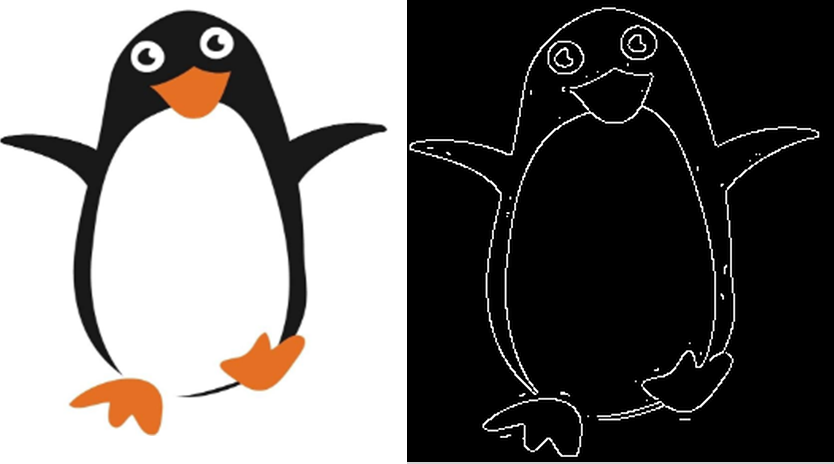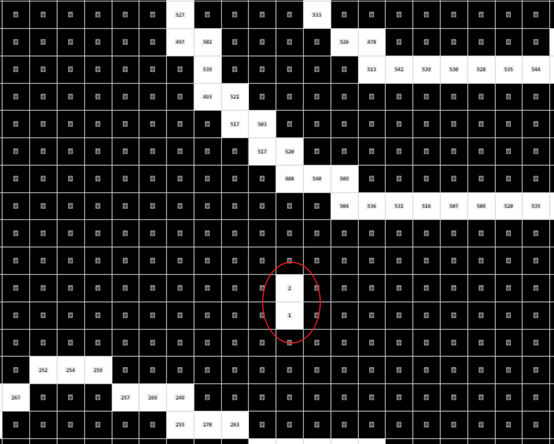使用双阈值限定后如下图，内部点消失了。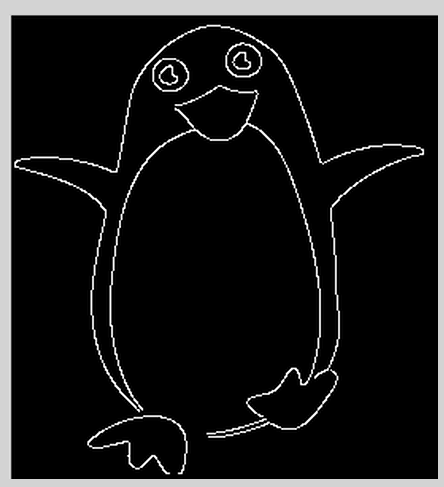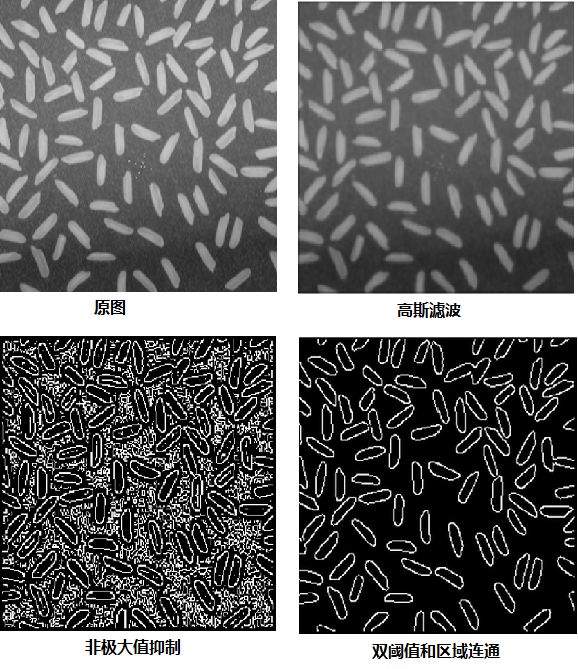##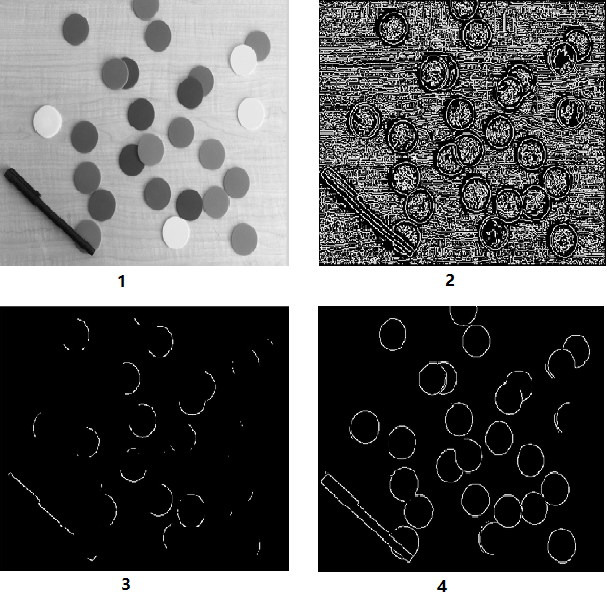5、参考文献

1、《数字图像处理与机器视觉》，第二版。 张铮、徐超、任淑霞、韩海玲等编著。

2、Canny 边缘检测

http://www.opencv.org.cn/opencvdoc/2.3.2/html/doc/tutorials/imgproc/imgtrans/canny_detector/canny_detector.html

3、Sobel算子的数学基础

http://blog.sciencenet.cn/blog-425437-1139187.html

4、Canny边缘检测

https://www.cnblogs.com/mmmmc/p/10524640.html

5、Canny算子中的非极大值抑制（Non-Maximum Suppression）分析

https://blog.csdn.net/kezunhai/article/details/11620357

6、一种改进非极大值抑制的Canny边缘检测算法

https://www.doc88.com/p-5174766661571.html### 作者的其它热门文章

0
0 收藏

0 评论
0 收藏
0